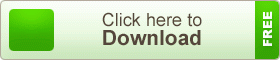# SSC Higher Mathematics Note 11th Chapter Coordinate Geometry

SSC Higher Mathematics Note 11th Chapter Coordinate Geometry. The portion of geometry where the algebraic expressions of points, straight lines, and curved lines are studied is familiar as the Coordinate Geometry. This portion of geometry is also known as the Analytic Geometry. With the plotting of points on the plane, the straight lines or the curved lines or the figures of the geometric regions made by them such as the triangles, the quadrilaterals, the circles etc. are expressed. The system of plotting of points on the plane is initiated by a French mathematician Rene Descartes (known as Descartes). The coordinate system of geometry initiated by Descartes is called after his name the Cartesian coordinate system. The coordinate geometry and analytical geometry are mainly based on the Cartesian coordinate system. So, Descartes is called the initiator of the analytical geometry.

## SSC Higher Mathematics Note 11th Chapter Coordinate GeometryIn  the first part of the chapter, through developing the concept of the Cartesian coordinate system among the learners, the tricks of determining the distance between the two points will be discussed. In the second part, the method of determining the measurement of any triangle, quadrilateral created by the straight line will be discussed and in the third part, the tricks of determining the slope of the straight line and determining the simplification of the joining straight line between the two points will be explained. No figure or equation associated with the curved line will be discussed here. In higher classes, it will be discussed elaborately.

teachingbd24.com is such a website where you will get all kinds of necessary information regarding educational notes, suggestions and question patterns of schools, colleges, and madrasas. Particularly, you will get here special notes of physics that will be immensely useful to both students and teachers. The builder of the website is Mr. Md. Shah Jamal who has been serving for 33 years as an Assistant Professor of Physics at BAF Shaheen College Dhaka. He expects that this website will meet up all the needs of Bengali version learners /students. He has requested concerned students and teachers to spread this website home and abroad.

SSC Higher Mathematics Note